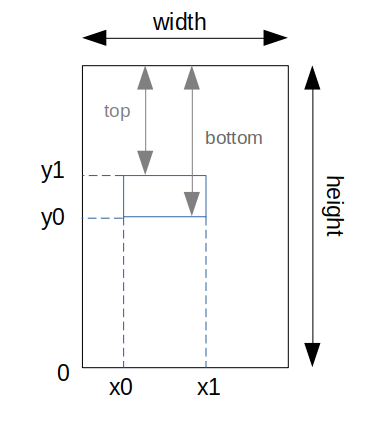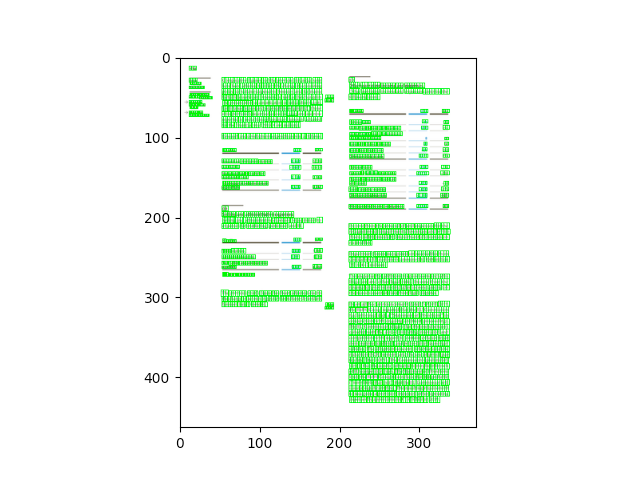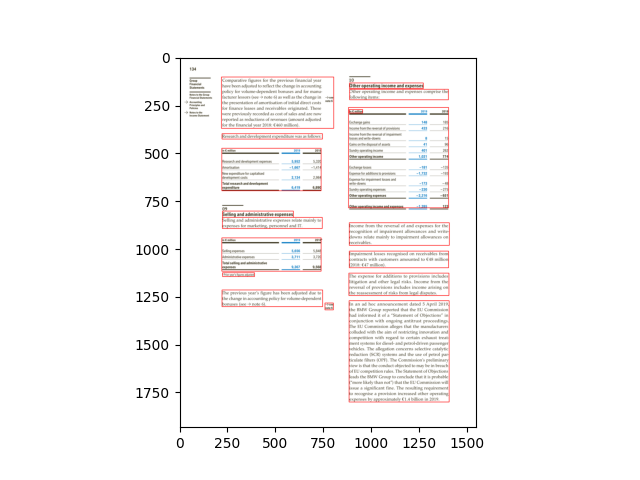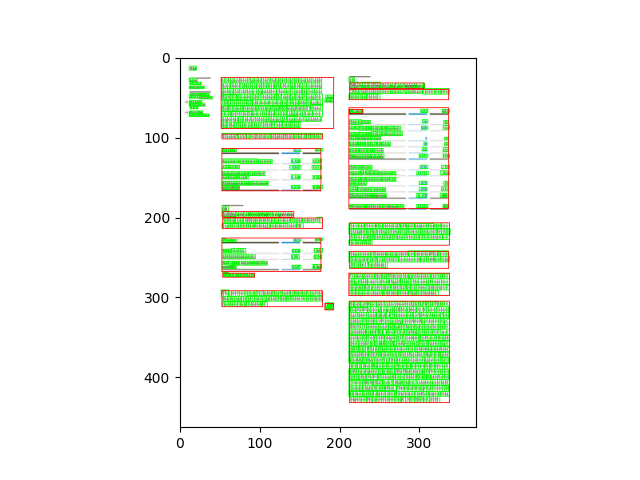# Coordinates system¶

The size of a page of a document can be obtained in the document object. The format is [width, height].

• ‘original_size’ corresponds to the size of the of the uploaded document (which can be a PDF file or an image.). The bounding boxes of the annotations are based on this size.

E.g.: original_size = [372.48, 463.68]

```document.pages[i]['original_size']
```
• “size” is the dimension of the image representation of a document page. These representations are used for computer vision tasks and the SmartView. E.g.: size = [1552, 1932]

```document.pages[i]['size']
```

The coordinates system used has its origin in the bottom left corner of the page.To visualize the character bounding boxes of a document and overlapping them in the image opened with the python library PIL, for example, we can resize the image to the size in which they are based (original_size). The following code can be used for this:

```doc_bbox = document.get_bbox()
image_path = document.image_paths[i]

size = document.pages[i]['size']
original_size = document.pages[i]['original_size']

factor_x = original_size / size
factor_y = original_size / size

image = Image.open(image_path).convert('RGB')
image.thumbnail((int(image.size * factor_x), int(image.size * factor_y)), Image.ANTIALIAS)
height = image.size

image_characters_bbox = [char_bbox for _, char_bbox in doc_bbox.items() if char_bbox["page_number"] - 1 == i]

for bbox in image_characters_bbox:
pp1 = (int(bbox["x0"]), int((height - bbox["y0"])))
pp2 = (int(bbox["x1"]), int((height - bbox["y1"])))
image = cv2.rectangle(np.array(image), pp1, pp2, (0, 255, 0), 1)

plt.imshow(image)
plt.show()

# Note: cv2 has the origin of the y coordinates in the upper left corner. Therefore, for visualization, the height of the
# image is subtracted to the y coordinates.
```The coordinates obtained from the segmentation endpoint of the API are based in the image array shape. To visualize the segmentation bounding boxes of a page on an image opened with the python library PIL, for example, we can overlap them directly.

```image_path = document.image_paths[i]
image = Image.open(image_path).convert('RGB')
image_segmentation_bboxes = get_results_from_segmentation(document.id, project_id)

for bbox in image_segmentation_bboxes[i]:
pp1 = (int(bbox["x0"]), int(bbox["y0"]))
pp2 = (int(bbox["x1"]), int(bbox["y1"]))
image = cv2.rectangle(np.array(image), pp1, pp2, (255, 0, 0), 1)

plt.imshow(image)
plt.show()
```To visualize both at the same time we can convert the coordinates from the segmentation result to be based on the image size used for the characters bbox.

```doc_bbox = document.get_bbox()
image_path = document.image_paths[i]

factor_x = original_size / size
factor_y = original_size / size

image = Image.open(image_path).convert('RGB')
image_factor = image.copy()

image.thumbnail((int(image.size * factor_x), int(image.size * factor_y)), Image.ANTIALIAS)
height = image.size

image_characters_bbox = [char_bbox for _, char_bbox in doc_bbox.items() if char_bbox["page_number"] - 1 == i]

for bbox in image_characters_bbox:
pp1 = (int(bbox["x0"]), int((height - bbox["y0"])))
pp2 = (int(bbox["x1"]), int((height - bbox["y1"])))
image = cv2.rectangle(np.array(image), pp1, pp2, (0, 255, 0), 1)

image_segmentation_bboxes = get_results_from_segmentation(document.id, project_id)

for bbox in image_segmentation_bboxes[i]:
pp1 = (int(bbox["x0"] * factor_x), int(bbox["y0"] * factor_y))
pp2 = (int(bbox["x1"] * factor_x), int(bbox["y1"] * factor_y))
image = cv2.rectangle(np.array(image), pp1, pp2, (255, 0, 0), 1)

plt.imshow(image)
plt.show()
```